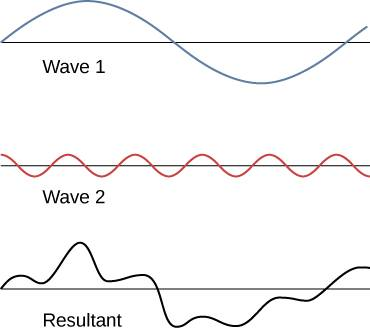# Constructive interference of harmonic electromagnetic waves

• I
MartinG
Hello !

I have a doubt as to how is this case, if it occurs, of the constructive interference of two harmonic electromagnetic waves but of different wavelengths or frequencies between them.

That is, if between the two electromagnetic waves a new and unique electromagnetic wave is created and what characteristics would this new created wave have, such as its frequency, its amplitude and its final or resulting energy.

I thank you for your answers and I send you my regards.

2022 Award
You can superpose waves of different frequencies, yes. Electromagnetism is a linear theory so you may simply add amplitudes. The resulting wave cannot be characterised by a single frequency, much as a chord is not a single note.

Last edited:
•MartinG and PeroK
MartinG
You can superpose waves of different frequencies, yes. Electromagnetism is a linear theory so you may simply add amplitudes. The resulting wave cannot be characterised by a single frequency, much as a chord is not a single note.

I will ask you again to see if I understood correctly.

In the superposition of electromagnetic waves, even if they are harmonic waves but of different frequencies, from the two waves a single electromagnetic wave different from the other two would not be formed, but rather we would have a case as shown in the figure I placed, where the resulting wave would it be like the third wave shown in the figure?2022 Award
If your third figure is the sum of the first two, then yes.

•MartinG
Hello !

I have a doubt as to how is this case, if it occurs, of the constructive interference of two harmonic electromagnetic waves but of different wavelengths or frequencies between them.

That is, if between the two electromagnetic waves a new and unique electromagnetic wave is created and what characteristics would this new created wave have, such as its frequency, its amplitude and its final or resulting energy.

I thank you for your answers and I send you my regards.
It depends on whether or not the two waves are mutually coherent. For example, the output of two different (independent) lasers cannot be combined to produce interference.

••MartinG, berkeman, vanhees71 and 1 other person
•